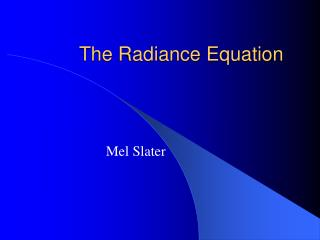DownloadDownload PresentationTélécharger la présentation- - - - - - - - - - - - - - - - - - - - - - - - - - - E N D - - - - - - - - - - - - - - - - - - - - - - - - - - -
##### Presentation Transcript

1. The Radiance Equation Mel Slater

2. Outline • Introduction • Light • Simplifying Assumptions • Radiance • Reflectance • The Radiance Equation • Traditional Rendering Solutions • Visibility • Conclusions

3. Introduction • Lighting is the central problem of real-time graphics rendering • Arbitrary shaped lights • Changes in lighting conditions • Real-time shadows • Real-time reflections • Mixtures of many different types of surface

4. Introduction • Real-time walkthrough with global illumination • Possible under limited conditions • Radiosity (diffuse surfaces only) • Real-time interaction • Not possible except for special case local illumination • Why is the problem so hard?

5. Light • Visible light is electromagnetic radiation with wavelengths approximately in the range from 400nm to 700nm 400nm 700nm

6. Light: Photons • Light can be viewed as wave or particle phenomenon • Particles are photons • packets of energy which travel in a straight line in vaccuum with velocity c (300,000m.p.s.) • The problem of how light interacts with surfaces in a volume of space is an example of a transport problem.

7. Light: Radiant Power •  denotes the radiant energy or flux in a volume V. • The flux is the rate of energy flowing through a surface per unit time (watts). • The energy is proportional to the particle flow, since each photon carries energy. • The flux may be thought of as the flow of photons per unit time.

8. Light: Flux Equilibrium • Total flux in a volume in dynamic equilibrium • Particles are flowing • Distribution is constant • Conservation of energy • Total energy input into the volume = total energy that is output by or absorbed by matter within the volume.

9. Light: Fundamental Equation • Input • Emission – emitted from within volume • Inscattering – flows from outside • Output • Streaming – without interaction with matter in the volume • Outscattering – reflected out from matter • Absorption – by matter within the volume • Input = Output

10. Light: Equation • (p,) denotes flux at pV, in direction  • It is possible to write down an integral equation for (p,) based on: • Emission+Inscattering = Streaming+Outscattering + Absorption • Complete knowledge of (p,) provides a complete solution to the graphics rendering problem. • Rendering is about solving for (p,).

11. Simplifying Assumptions • Wavelength independence • No interaction between wavelengths (no fluorescence) • Time invariance • Solution remains valid over time unless scene changes (no phosphorescence) • Light transports in a vacuum (non-participating medium) – • ‘free space’ – interaction only occurs at the surfaces of objects

12. Radiance • Radiance (L) is the flux that leaves a surface, per unit projected area of the surface, per unit solid angle of direction. n d = L dA cos d L  dA

13. n d L  dA Radiance • For computer graphics the basic particle is not the photon and the energy it carries but the ray and its associated radiance. Radiance is constant along a ray.

14. Radiance: Radiosity, Irradiance • Radiosity - is the flux per unit area that radiates from a surface, denoted by B. • d = B dA • Irradiance is the flux per unit area that arrives at a surface, denoted by E. • d = E dA

15. Radiosity and Irradiance • L(p,) is radiance at p in direction  • E(p,) is irradiance at p in direction  • E(p,) = (d/dA) = L(p,) cos d

16. f(p, i ,r ) i r Reflected ray Incident ray Illumination hemisphere Reflectance • BRDF • Bi-directional • Reflectance • Distribution • Function • Relates • Reflected radiance to incoming irradiance

17. Reflectance: BRDF • Reflected Radiance = BRDFIrradiance • L(p, r ) = f(p,i ,r ) E(p, i ) • = f(p,i ,r ) L(p, i ) cosi di • In practice BRDF’s hard to specify • Rely on ideal types • Perfectly diffuse reflection • Perfectly specular reflection • Glossy reflection • BRDFs taken as additive mixture of these

19. The Radiance Equation: Reflection • Total reflected radiance in direction : • f(p,i ,) L(p, i ) cosi di • Radiance Equation: • L(p,  ) = Le(p,  ) +  f(p,i ,) L(p, i ) cosi di • (Integration over the illumination hemisphere)

20. The Radiance Equation • p is considered to be on a surface, but can be anywhere, since radiance is constant along a ray, trace back until surface is reached at p’, then • L(p, i) = L(p’, i) L(p, ) depends on allL(p*, i) which in turnare recursively defined. p* i L(p,  ) p The radiance equation models global illumination.

21. Traditional Solutions to the Radiance Equation • The radiance equation embodies totality of all 2D projections (view). • Extraction of a 2D projection to form an image is called rendering.